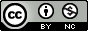Araştırma Makalesi

### Reciprocal Complementary Distance Energy of Complement of Line Graphs of Regular Graphs

Yıl 2021, Cilt: 9 Sayı: 1, 36 - 41, 01.03.2021

### Öz

The reciprocal complementary distance ($RCD$) matrix of a graph $G$ is defined as $RCD(G) = [r_{ij}]$, where $r_{ij} = \frac{1}{1+D-d_{ij}}$ if $i \neq j$ and $r_{ij} = 0$, otherwise, where $D$ is the diameter of $G$ and $d_{ij}$ is the distance between the vertices $v_i$ and $v_j$ in $G$. The $RCD$-energy of $G$ is defined as the sum of the absolute values of the eigenvalues of $RCD$-matrix. Two graphs are said to be $RCD$-equienergetic if they have same $RCD$-energy. In this paper, the $RCD$-energy of the complement of line graphs of certain regular graphs in terms of the order and degree is obtained and as a consequence, pairs of $RCD$-equienergetic graphs of same order and having different $RCD$-eigenvalues are constructed.

### Destekleyen Kurum

University Grants Commission (UGC), New Delhi

### Teşekkür

The author HSR is thankful to the University Grants Commission (UGC), New Delhi, for support through UGC-SAP DRS-III, 2016-2021: F.510/3/ DRS-III /2016 (SAP-I).

### Kaynakça

•  Buckley, F.: Iterated line graphs. Congr. Numer. 33, 390–394 (1981).
•  Buckley, F.: The size of iterated line graphs. Graph Theory Notes of New York. 25, 33–36 (1993).
•  Cvetkovic, D., Rowlinson, P., Simic, S.: Introduction to the Theory of Graph Spectra. Cambridge University Press. Cambridge (2010).
•  Gutman, I.: The energy of a graph. Ber. Math. Stat. Sekt. Forschungsz. Graz. 103, 1–22 (1978).
•  Ivanciuc, O., Ivanciuc, T., Balaban, A. T.: The complementary distance matrix, a new moleculargraph metric. ACHModels Chem. 137, 57–82 (2000).
•  Indulal, G.: D-spectrum and D-energy of complements of iterated line graphs of regular graphs. J. Alg. Stru. Appl. 4, 51–56 (2017). https://doi.org/10.29252/asta.4.1.51
•  Jenežic, D., Milicevic, A., Nikolic, S., Trinajstic, N.: Graph Theoretical Matrices in Chemistry. University of Kragujevac. Kragujevac (2007). https://doi.org/10.1021/ci700278s
•  Li, X., Shi, Y., Gutman, I.: Graph Energy. Springer. New York (2012). https://doi.org/10.1007/978-1-4614-4220-2
•  Ramane, H. S., Gudodagi, G. A.: Reciprocal complementary equienergetic graphs. Asian-European J. Math. 9, ID: 1650084, pages 15 (2016). https://doi.org/10.1142/S1793557116500844
Yıl 2021, Cilt: 9 Sayı: 1, 36 - 41, 01.03.2021

### Kaynakça

•  Buckley, F.: Iterated line graphs. Congr. Numer. 33, 390–394 (1981).
•  Buckley, F.: The size of iterated line graphs. Graph Theory Notes of New York. 25, 33–36 (1993).
•  Cvetkovic, D., Rowlinson, P., Simic, S.: Introduction to the Theory of Graph Spectra. Cambridge University Press. Cambridge (2010).
•  Gutman, I.: The energy of a graph. Ber. Math. Stat. Sekt. Forschungsz. Graz. 103, 1–22 (1978).
•  Ivanciuc, O., Ivanciuc, T., Balaban, A. T.: The complementary distance matrix, a new moleculargraph metric. ACHModels Chem. 137, 57–82 (2000).
•  Indulal, G.: D-spectrum and D-energy of complements of iterated line graphs of regular graphs. J. Alg. Stru. Appl. 4, 51–56 (2017). https://doi.org/10.29252/asta.4.1.51
•  Jenežic, D., Milicevic, A., Nikolic, S., Trinajstic, N.: Graph Theoretical Matrices in Chemistry. University of Kragujevac. Kragujevac (2007). https://doi.org/10.1021/ci700278s
•  Li, X., Shi, Y., Gutman, I.: Graph Energy. Springer. New York (2012). https://doi.org/10.1007/978-1-4614-4220-2
•  Ramane, H. S., Gudodagi, G. A.: Reciprocal complementary equienergetic graphs. Asian-European J. Math. 9, ID: 1650084, pages 15 (2016). https://doi.org/10.1142/S1793557116500844

### Ayrıntılar

Birincil Dil İngilizce Matematik Articles Harishchandra RAMANE Karnatak University, Dharwad 0000-0003-3122-1669 India B PARVATHALU Bu kişi benim Karnatak Arts College, Dharwad 0000-0002-5151-8446 India F.510/3/ DRS-III /2016 (SAP-I) 1 Mart 2021 1 Kasım 2019 9 Kasım 2020 Yıl 2021 Cilt: 9 Sayı: 1

### Kaynak Göster

 Bibtex @araştırma makalesi { mathenot641660, journal = {Mathematical Sciences and Applications E-Notes}, eissn = {2147-6268}, address = {}, publisher = {Murat TOSUN}, year = {2021}, volume = {9}, number = {1}, pages = {36 - 41}, doi = {10.36753/mathenot.641660}, title = {Reciprocal Complementary Distance Energy of Complement of Line Graphs of Regular Graphs}, key = {cite}, author = {Ramane, Harishchandra and Parvathalu, B} } APA Ramane, H. & Parvathalu, B. (2021). Reciprocal Complementary Distance Energy of Complement of Line Graphs of Regular Graphs . Mathematical Sciences and Applications E-Notes , 9 (1) , 36-41 . DOI: 10.36753/mathenot.641660 MLA Ramane, H. , Parvathalu, B. "Reciprocal Complementary Distance Energy of Complement of Line Graphs of Regular Graphs" . Mathematical Sciences and Applications E-Notes 9 (2021 ): 36-41 Chicago Ramane, H. , Parvathalu, B. "Reciprocal Complementary Distance Energy of Complement of Line Graphs of Regular Graphs". Mathematical Sciences and Applications E-Notes 9 (2021 ): 36-41 RIS TY - JOUR T1 - Reciprocal Complementary Distance Energy of Complement of Line Graphs of Regular Graphs AU - HarishchandraRamane, BParvathalu Y1 - 2021 PY - 2021 N1 - doi: 10.36753/mathenot.641660 DO - 10.36753/mathenot.641660 T2 - Mathematical Sciences and Applications E-Notes JF - Journal JO - JOR SP - 36 EP - 41 VL - 9 IS - 1 SN - -2147-6268 M3 - doi: 10.36753/mathenot.641660 UR - https://doi.org/10.36753/mathenot.641660 Y2 - 2020 ER - EndNote %0 Mathematical Sciences and Applications E-Notes Reciprocal Complementary Distance Energy of Complement of Line Graphs of Regular Graphs %A Harishchandra Ramane , B Parvathalu %T Reciprocal Complementary Distance Energy of Complement of Line Graphs of Regular Graphs %D 2021 %J Mathematical Sciences and Applications E-Notes %P -2147-6268 %V 9 %N 1 %R doi: 10.36753/mathenot.641660 %U 10.36753/mathenot.641660 ISNAD Ramane, Harishchandra , Parvathalu, B . "Reciprocal Complementary Distance Energy of Complement of Line Graphs of Regular Graphs". Mathematical Sciences and Applications E-Notes 9 / 1 (Mart 2021): 36-41 . https://doi.org/10.36753/mathenot.641660 AMA Ramane H. , Parvathalu B. Reciprocal Complementary Distance Energy of Complement of Line Graphs of Regular Graphs. Math. Sci. Appl. E-Notes. 2021; 9(1): 36-41. Vancouver Ramane H. , Parvathalu B. Reciprocal Complementary Distance Energy of Complement of Line Graphs of Regular Graphs. Mathematical Sciences and Applications E-Notes. 2021; 9(1): 36-41. IEEE H. Ramane ve B. Parvathalu , "Reciprocal Complementary Distance Energy of Complement of Line Graphs of Regular Graphs", Mathematical Sciences and Applications E-Notes, c. 9, sayı. 1, ss. 36-41, Mar. 2021, doi:10.36753/mathenot.641660• Makale Dosyaları